9. MODELS OF ATOMIC NUCLEI OF CHEMICAL ELEMENTS

9.1. General Data on Atomic Nuclei

If the electromagnetic models of the proton and the neutron were known, the task of the determination of the structure of the atomic nuclei of chemical elements would be simplified significantly. But they are not available. That’s why we should base our assertions only on the experimental facts and deductions, which originate from the results of theoretical investigations , , , , , , .

The first and the most important result of our investigations, which belongs to the atomic nucleus, is the lack of orbital movement of the electron in the atom. The electrons interact with the atomic nuclei with their axes of rotation. It is possible if the protons of the nucleus are arranged on its surface , , .

Thus, in order to provide the interaction of each electron with the nucleus, it is necessary to arrange the protons on the surface of the nucleus. The same charges of the protons exclude the structure of the nucleus, in which the protons could touch each other. The nature builds the nucleus in such a way that a neutron should be between the protons. As the last requirement is difficult to be met  when there are many protons in the nucleus, additional neutrons are attracted. That’s why the nuclei of almost all chemical elements contain more neutrons than protons. When the number of the protons and the neutrons in the nucleus is increased, the share of “extra” neutrons is increased. It is clear, because it is impossible to get geometrical symmetry of the nucleus, in which the neutrons should be present between the protons , .

It seems that the neutrons are penetrable for magnetic fields of the protons and unpenetrable or weakly penetrable for their electrical fields. Screening the like electrical fields of the protons, the neutrons create the conditions when the magnetic poles of the protons interact with the unlike magnetic poles of the neutrons .

The electron and the proton have the charges and the magnetic moments. We have already found that the magnetic field of the electron is similar to the magnetic field of a bar cylindrical magnet. One can suppose that the charge of the proton helps to form the same magnetic field as the field of the electron, i.e. the structure of the magnetic field of the proton is similar to the structure of the magnetic field of the bar cylindrical magnet. Let us call such magnetic field a simple magnetic field .

The neutron has magnetic moment as well and, consequently, magnetic field. But we know nothing about the structure of its magnetic field. If it is similar to magnetic field of the bar cylindrical magnet, the proton and the neutron are connected as the bar magnets, and the structure of the nuclei should be linear. If the neutron has a compound magnetic field, which consists of several magnetic poles, the construction of the atomic nuclei with more complicated space configuration is possible .

That’s why the determination of the structure of the magnetic field of the neutron is one of the priority tasks in our search. We have already called the magnetic field of the proton, which is similar to the magnetic field of the bar cylindrical magnet, a simple symmetrical magnetic field. There is every reason to believe that the magnetic field of the neutron has more complicated configuration; that’s why let us call it a compound magnetic field .

As the nuclei of all chemical elements consist of the positively charged protons and uncharged neutrons, it is necessary to systematize the information concerning the main characteristics of these elementary particles in order to detail the principles, which control the formation of the nuclei of chemical elements.

9.2. On Model of the Proton

It is known that rest mass of the proton is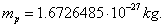Electrical charge of the proton is positive and is equal to the negative charge of the electron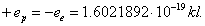. Magnetic moment of the proton is equal to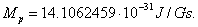Electromagnetic structure of the proton is unknown. If we suppose that it (as the electron) has the form of a torus, radius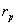of this torus is as follows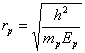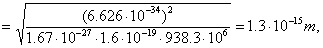(230)

where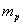is mass of the proton,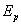is the photon energy of the proton.

Thus, radius of the proton is by three orders of magnitude less than radius of the electron. Spin of the proton (as spin of the electron) is equal to Planck’s constant. Vectors of these spins are directed along the axes of their rotation. The directions of spins both of the proton and the electron coincide with the directions of vectors of their magnetic moments . It appears from the formula (149), which connects Planck’s constant and magnetic moment of  electron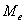. Let us present it in the following way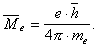(231)

In modern physics, vectors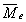and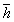are considered to be opposite directional. It is reasoned by the fact that the charge of the electron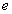in the formula (149) is negative. Rather a strange reason. The nature gives vector properties to values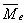and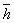. Physicists themselves have given a negative sign to the charge of the electron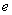. It is conventional agreement, not the law of the nature! In formula (149), the charge of the electronis a scalar value, and we have no reason to write it as follows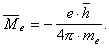(232)

Let alone logic of modern physicists; we’d like to consider the formula (231), from which it appears that vectorsand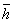are directed to one side.

Magnetic field strength in the vicinity of geometrical centre of the toroidal model of the proton is considerably greater than the same magnetic field strength of the electron. If we assume that the proton is a torus as well, we’ll get magnetic field strength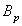in the vicinity of the centre of symmetry of the proton using magnetic moment of the proton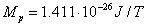and its photon energy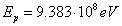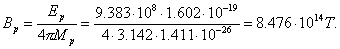(233)

This is colossal magnetic field strength in the centre of symmetry of the proton. Outside this centre, it is decreased quickly. Along the axis of rotation of the proton this decrease is inversely proportional to cube of the distance from its geometrical centre . Such large magnetic field strength generates corresponding large magnetic forces. If magnetic field of the proton is similar to magnetic field of the bar cylindrical magnet, unlike magnetic poles will juxtapose the protons and the neutrons.

If we imagine the proton in the form of a sphere with radius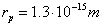, Coulomb force will act during the direct contact of two protons between them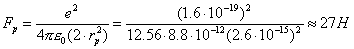(234)

For the sake of comparison, let us calculate gravitational force, which exists between the protons in this case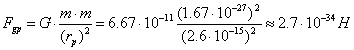(235)

The results of these calculations prove that during formation of the atomic nuclei electrostatic forces and magnetic ones, not gravitational forces, play a decisive role. They form the atomic nuclei.

In order to get a clear understanding on the proton model, we’d like to note that in the first approximation it is a rotating torus, which can be presented in the form of a geometrical figure having a form of an apple with the magnetic field lines passing along the axis of the apple and closing on each other. Electrical field lines are directed perpendicular to the magnetic filed lines or perpendicular to toroidal surface. Such model has almost spherical electrical field and two magnetic poles: the north pole and the south one. The poles are formed at different ends of axis of rotation of torus. Let us call the described structure of magnetic field a simple structure, and the unknown structure of electromagnetic field of the neutron a compound structure .

9.3. On Model of the Neutron

It is known that rest mass of the neutron is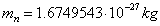. The difference between mass of the neutron and the proton is equal to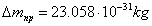. Thus, mass of the neutron is larger than mass of the proton by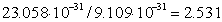masses of the electron. It appears from this that in order a proton becomes a neutron, it should capture 2.531 electrons. As there are no electrons with fractional mass, the proton should absorb the whole number of the electrons. If it absorbs three electrons, and its mass is increased only by 2.531 masses of the electron, a question arises: what will become of the remainder of mass of the electron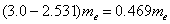? Modern physics explains the disturbed balance of masses in this process simply: a neutrino is born, which has no charge; that’s why it is very difficult to register the birth of this particle as it is assumed in modern physics. The neutron has magnetic field. Its magnetic moment is estimated by the value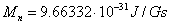.

The neutron has no charge. As the mass of the neutron differs insignificantly from the mass of the proton, one can suppose that they have similar geometrical dimensions. One can suppose that the neutron radius is almost the same as the proton radius or is a bit larger than the proton radius.

As we try to find the principle, by which the nature is guided when it forms the atomic nuclei, our information concerning the models of the proton and neutron shows that these particles, which control the formation of the atomic nuclei, have the following properties: the charge and magnetic moment of the proton as well as magnetic moment of the neutron and the lack of charge. Availability of magnetic moments with these particles gives us the reason to imagine that they have magnetic poles .

Magnetic forces of unlike poles of magnetic fields of the proton and the neutron are the only forces, which can connect these particles with each other. Electrostatic forces of the protons are the only forces, which limit the approach of the protons in the nucleus.

Nevertheless, the experiments have revealed the existence of nuclear forces connecting the protons and the neutrons in the atomic nuclei. The value of these forces is by a factor of 102 of electrostatic repulsive forces of the protons. The forces generating such interaction are called nuclei forces. Their nature remains unknown.

If we take into consideration large magnetic field strength near the centre of symmetry of the proton (233) and suppose that it is the same with the neutron, there is every reason to believe that magnetic forces of the proton and the neutron acting at the distances near to their geometrical centres are the forces, which have been called the nuclei ones .

Thus, we have a possibility to suppose that the nuclei forces are the magnetic forces acting at the smallest distances between the centres of masses of the protons and the neutrons. First of all, let us consider the spherical models of the protons and the neutrons with the magnetic fields, which are similar to symmetrical magnetic fields of the bar cylindrical magnets and the reasons of existence of six-pole magnetic with the neutron.

The Foundations of Physchemistry of Microworld

Ó2003 Kanarev Ph. M.

Internet Version - http://book.physchemistry.innoplaza.net

<< Back to Physchemistry Book Index

 Let us remind that 1TL=104 Gs